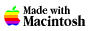Tue Nov 12 01:28:09 2019 GMTHome

Safety First

What's A "Water Rocket"?

Water-Rocket Simulation

### Q:  What do the simulation program variables mean and how do I measure and find them?

A:  Our simulation program has 12 parameters or variables that you can adjust to describe your rocket, and to control how the simulation runs.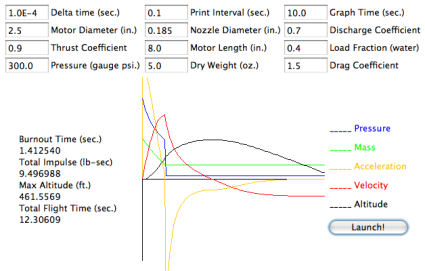### Rocket Parameters

Set the following input parameters to describe the rocket design you want to simulate. These parameters are then used by the simulator to calculate how your rocket will perform:

#### Motor Diameter

This is the diameter of the rocket motor, in inches. A 2-liter bottle motor has a diameter of 4.125 inches. The simulation assumes that the motor diameter and the rocket diameter are the same for the purpose of calculating drag during flight.

#### Motor Length

This is the length of the motor in inches. A 2-liter bottle motor has an approximate length of 9 inches. 4.125 diameter by 9 inches long is equivalent to 1.97 liters.

#### Nozzle Diameter

This is the inside diameter of the motor nozzle in inches. For a 2-liter bottle motor this is 0.84 inches.

Load Fraction is the fraction of the rocket motor volume that is filled with water at launch.

• A rocket half full of water has a load fraction of 0.5.
• A rocket one third full has a load fraction of 0.33
• A rocket one quarter full has a load fraction of 0.25
• Load Fraction is the ratio of the water volume to the total volume of the motor. For example, if you put 0.8 liters of water in a 2-liter motor the load fraction is 0.4.

#### Pressure

This is the gauge pressure inside the motor at launch in pounds per square inch. Typical values for launching plastic bottles range from 30 to 120 psi. Metal-can motors have been fired at 300 psi. Your bicycle-pump or compressor gauge will show this pressure.

#### Dry Weight

This is the weight of the complete rocket without water in ounces. Use your kitchen scale to measure the dry weight.

#### Drag Coefficient

The drag coefficient describes how easily your rocket moves through the air. It is used to calculate the force due to drag that slows a rocket during flight: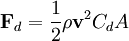Typical values for rockets range from 0.3 to 1.6 depending on the shape.

An eliptical nosed rocket would be on the low end of the range, and a relatively flat nosed rocket would be at the high end.

Typical is probably 0.8 for a cone of the design used on my example rocket.

### Simulation Parameters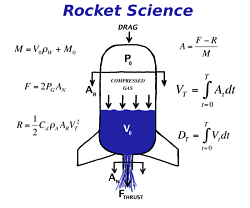The following parameters control how the simulation calculates the rocket performance. Changing these parameters will not be required for most uses of the simulation.

#### Delta Time

The simulation calculates the performance of the water rocket by integrating a coupled set of ordinary differential equations. This integration is done by calculating finite differences over a prescribed time interval: Delta Time.

For typical pressures, nozzles diameters, and volumes used in water rockets the default value of 0.0001 seconds (100 microseconds) is sufficient. If your rocket is operating at higher pressures you may want to use a smaller Delta Time, perhaps as low as 0.00001 seconds.

#### Graph Time

This controls the length of the X axis (time) on the chart. Set this to match, or exceed, the Total Flight time displayed in text on the screen.

#### Print Interval

The simulation prints a table of performance values to the Java Console of your browser when it runs. The Print Interval controls how often the values are printed.

#### Discharge Coefficient

The Discharge Coefficient represents the effect of the nozzle behaviour that is non-ideal. The instantanueous velocity of water exiting the nozzle is multiplied by the Discharge Coefficient to account for effects that reduce the flow, like enertial contraction of the stream around sharp corners in the nozzle throat.

This is an empirically derived constant.

#### Thrust Coefficient

The Thrust Coefficient represents the loss of thrust to non-ideal behaviours in the nozzle. The instantaneous thrust is multipled by the Thrust Coefficient to account for losses due to turbulence and simplifications in the thrust calculation.

This is an empirically derived constant.

 FaceBook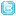TwitterDelicious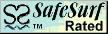Question? Comment? Send A Message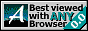updated 11-Dec-2009
3.233.226.151
Copyright © 1997-2012, TRD Associates LLC